# Ideals in residuated lattices

### Full PDFcarpathian_2021_37_1_53_63

Issue no:

The notion of ideal in residuated lattices is introduced in [Kengne, P. C., Koguep, B. B., Akume, D. and Lele, C.,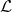-fuzzy ideals of residuated lattices, Discuss. Math. Gen. Algebra Appl., 39 (2019), No. 2, 181–201] and
[Liu, Y., Qin, Y., Qin, X. and Xu, Y.,  Ideals and
fuzzy ideals in residuated lattices, Int. J. Math. Learn \& Cyber., 8
(2017), 239–253] as a natural generalization of that of ideal in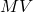algebras
(see [Cignoli, R., D’Ottaviano, I. M. L. and Mundici, D., Algebraic Foundations of many-valued Reasoning, Trends in Logic-Studia
Logica Library 7, Dordrecht: Kluwer Academic Publishers, 2000] and [Chang, C. C., Algebraic analysis of many-valued logic, Trans. Amer. Math. Soc., 88 (1958), 467–490]). If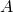is an MV algebra and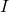is an ideal onthen the binary relation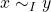iff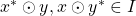, foris a congruence relation on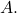In this
paper we find classes of residuated lattices for which the relation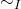(defined for MV algebras) is a congruence relation and we give new
characterizations for i-ideals and prime i-ideals in residuated lattices. As
a generalization of the case of BL algebras (see [Lele, C. and Nganou, J. B.,  MV-algebras derived from
ideals in BL-algebras, Fuzzy Sets and Systems, 218 (2013), 103–113]), we
investigate the relationship between i-ideals and deductive systems in
residuated lattices.